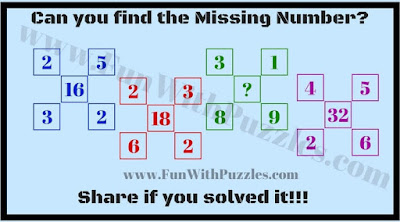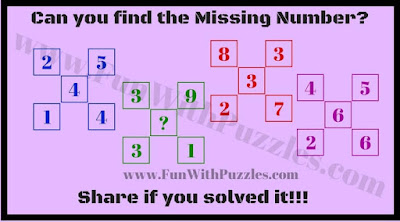After a series of Picture Brain Teasers, now it's time for Mathematical Brain Teasers. These Math Brain Teasers are related to the set of numbers that are related to each other with some Mathematical sequences or patterns. In these Puzzles, one number is missing in one of the sets which need to be calculated based upon Maths Pattern.
In each of these Math Brain Teasers, four sets of five numbers each are given. These five numbers in each set are linked with some mathematical pattern. Your challenge to find this Mathematical pattern and then find the missing number which will replace the question mark.
Answers to these Math Brain Teasers are given at the end. However, try to solve all these puzzles by yourself and then look at these answers to check the correctness of your answers.1. Easy Maths Brain Teaser2. Math Puzzles Brain Teaser3.Middle School Math Brain Teaser4. Maths Brain Teaser for adults5. Hard Maths Brain Teaser

Do checkout more Maths Brain Teasers as mentioned below which will test your brain
List of Brain Testing Maths Brain Teasers

Interesting and Confusing Maths Brain Test: This Math Brain Test Contains mixed Mathematical Brain Teasers to tickle your mind.

Test your Brain with Smart Questions: This Brain Test not only contains Maths Brain Teasers but also Logical and Picture Puzzles.

Brain Teasers and Riddles IQ Test: Again this mixed IQ Test contains all types of different brain teasers and riddles.

Mathematical Riddles for Teens to Complete the Series: These are the Mathematical Riddles in which series of numbers are given and your challenge is to calculate the next number in the series.

Answers to these Math Brain Teasers are given below
1. 21

2. 8

3. 75

4. 11

5. 4